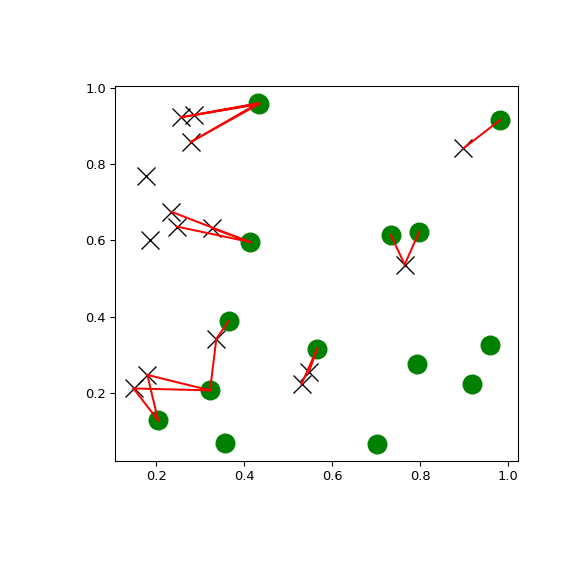# scipy.spatial.KDTree.query_ball_tree¶

KDTree.query_ball_tree(self, other, r, p=2.0, eps=0)[source]

Find all pairs of points between self and other whose distance is at most r

Parameters
otherKDTree instance

The tree containing points to search against.

rfloat

The maximum distance, has to be positive.

pfloat, optional

Which Minkowski norm to use. p has to meet the condition 1 <= p <= infinity.

epsfloat, optional

Approximate search. Branches of the tree are not explored if their nearest points are further than r/(1+eps), and branches are added in bulk if their furthest points are nearer than r * (1+eps). eps has to be non-negative.

Returns
resultslist of lists

For each element self.data[i] of this tree, results[i] is a list of the indices of its neighbors in other.data.

Examples

You can search all pairs of points between two kd-trees within a distance:

>>> import matplotlib.pyplot as plt
>>> import numpy as np
>>> from scipy.spatial import KDTree
>>> np.random.seed(21701)
>>> points1 = np.random.random((15, 2))
>>> points2 = np.random.random((15, 2))
>>> plt.figure(figsize=(6, 6))
>>> plt.plot(points1[:, 0], points1[:, 1], "xk", markersize=14)
>>> plt.plot(points2[:, 0], points2[:, 1], "og", markersize=14)
>>> kd_tree1 = KDTree(points1)
>>> kd_tree2 = KDTree(points2)
>>> indexes = kd_tree1.query_ball_tree(kd_tree2, r=0.2)
>>> for i in range(len(indexes)):
...     for j in indexes[i]:
...         plt.plot([points1[i, 0], points2[j, 0]],
...             [points1[i, 1], points2[j, 1]], "-r")
>>> plt.show()#### Previous topic

scipy.spatial.KDTree.query_ball_point

#### Next topic

scipy.spatial.KDTree.query_pairs